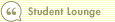Course 404: Putting DCF into Action
Step 3--Discount Projected Free Cash Flows to PresentIn this course1 Introduction 2 Step 1--Project Free Cash Flow 3 Step 2--Determine a Discount Rate 4 Step 3--Discount Projected Free Cash Flows to Present 5 Step 4--Calculate Discounted Perpetuity Value 6 Step 5--Add It All Up 7 The Bottom LineThe next step is to discount each of the individual year's cash flows to express them in terms of today's dollars. Remember we are using the following formula, and the "discount factor" just represents the denominator in the equation. We can then multiply each year's cash flow by the discount factor to get the present value of each cash flow.

Present Value of Cash Flow in Year N =
CF at Year N / (1 + R)^N

CF = Cash Flow
R = Required Return (Discount Rate), in this case 9%
N = Number of Years in the Future

Last Year: \$500.00
Year 1: 575.00 x (1 / 1.09^1) = 528
Year 2: 661.25 x (1 / 1.09^2) = 557
Year 3: 760.44 x (1 / 1.09^3) = 587
Year 4: 874.50 x (1 / 1.09^4) = 620
Year 5: 1005.68 x (1 / 1.09^5) = 654
Year 6: 1055.96 x (1 / 1.09^6) = 630
Year 7: 1108.76 x (1 / 1.09^7) = 607
Year 8: 1164.20 x (1 / 1.09^8) = 584
Year 9: 1222.41 x (1 / 1.09^9) = 563
Year 10: 1283.53 x (1 / 1.09^10) = 542

We then add up all the discounted cash flows from Years 1 through 10, and come up with a value of \$5,870 million (\$5.87 billion).Search Print Lesson  | FeedbackDel.icio.us |digg itLearn how to invest like a pro with Morningstar’s Investment Workbooks (John Wiley & Sons, 2004, 2005), available at online bookstores.© Copyright 2015 Morningstar, Inc. All rights reserved. Please read our Privacy Policy. If you have questions or comments please contact Morningstar.